# POISSON.DIST Function

Calculates the Poisson probability mass function

## WHAT is the POISSON.DIST Function?

The POISSON.DIST function is categorized under Excel Statistical functions. It will calculate the Poisson probability mass function.

As a financial analyst, POISSON.DIST is useful in forecasting revenue. Also, we can use it to predict the number of events occurring over a specific time, e.g., the number of cars arriving at the mall parking per minute.

The POISSON.DIST function was introduced in MS Excel 2010 and hence not available in earlier versions. For older versions of MS Excel, we can use the POISSON function.

### Formula

=POISSON.DIST(x,mean,cumulative)

The POISSON.DIST function uses the following arguments:

1. (required argument) – This is the number of events for which we want to calculate the probability. The value must be greater than or equal to 0.
2. Mean (required argument) – This is the expected number of events. The argument must be greater than or equal to zero.
3. Cumulative (required argument) – This is the logical argument that specifies the type of distribution to be calculated. It can either be:
• TRUE – Returns the cumulative Poisson probability that the number of random events occurring will be between zero and x inclusive.
• FALSE – Returns the Poisson probability mass function that the number of events occurring will be exactly x.

The Poisson probability mass function calculates the probability that there will be exactly x occurrences and is given by the formula: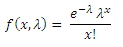Where λ is the expected number of occurrences within the specified time period.

The cumulative Poisson distribution function calculates the probability that there will be at most x occurrences and is given by the formula: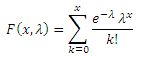### How to use the POISSON.DIST Function in Excel?

To understand the uses of the POISSON.DIST function, let’s consider an example:

#### Example

Suppose we are given the following data:

• Number of events: 5
• Expected mean: 10

To find out the Cumulative Poisson probability, we will use the following formula: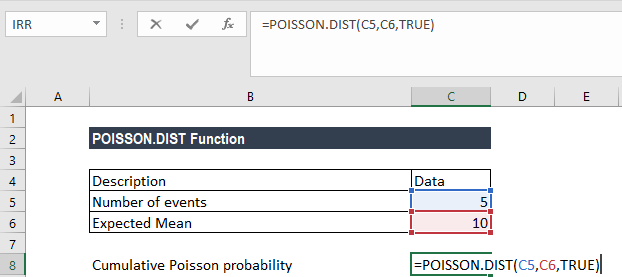We get the result below: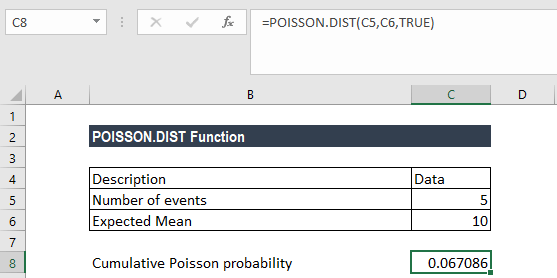To find out the Poisson probability mass function, we will use the following formula: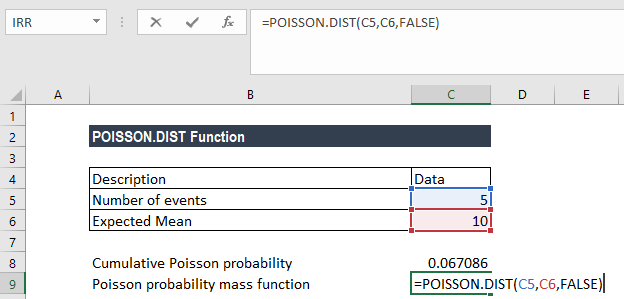We get the result below: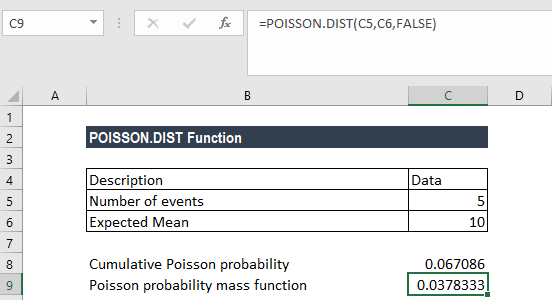### A few notes about the POISSON.DIST Function

1. #NUM! error – Occurs if either:
• The given value of x is less than zero; or
• The given value of mean is less than zero.
2. #VALUE! error – Occurs when any of the argument provided is non-numeric.
3. If size is not an integer, it is truncated.

Thanks for reading CFI’s guide to important Excel functions! By taking the time to learn and master these functions, you’ll significantly speed up your financial analysis. To learn more, check out these additional CFI resources:

• Excel Functions for Finance